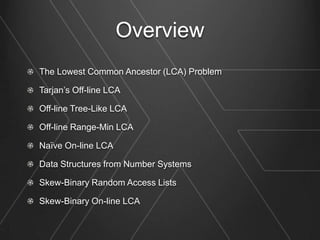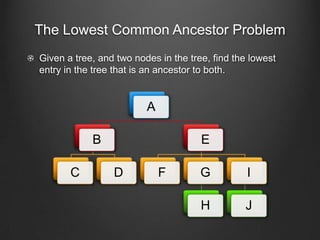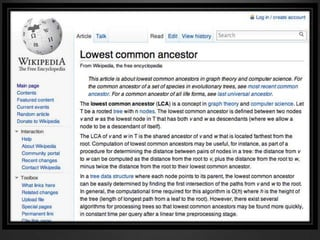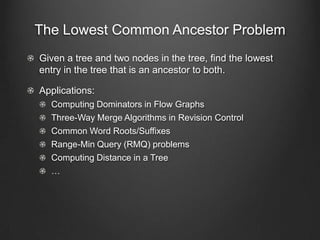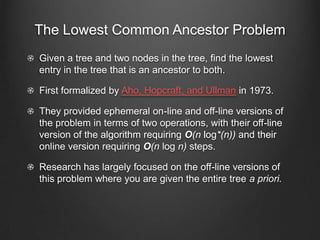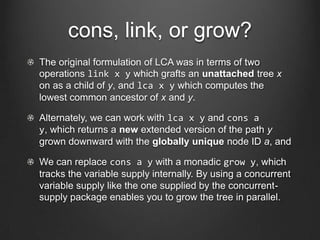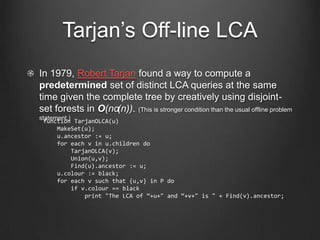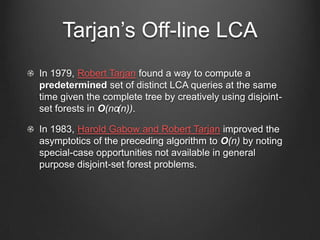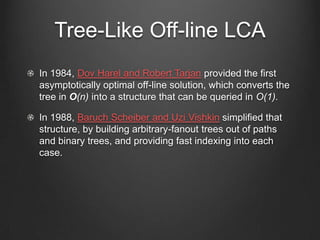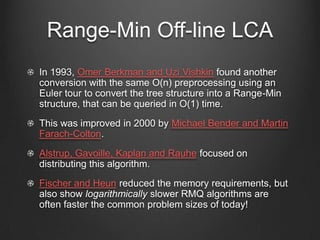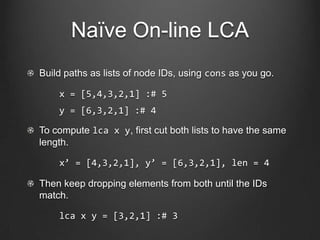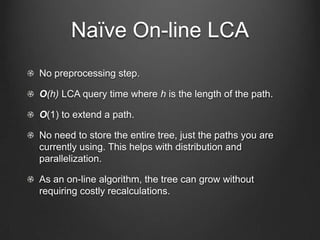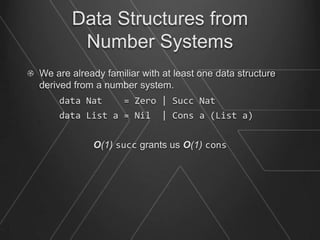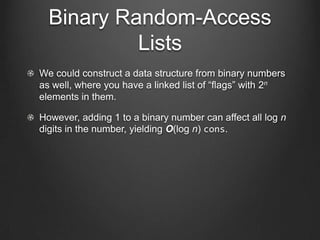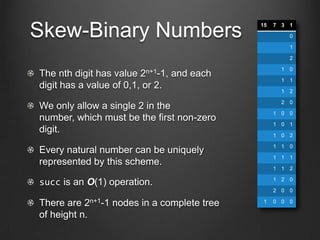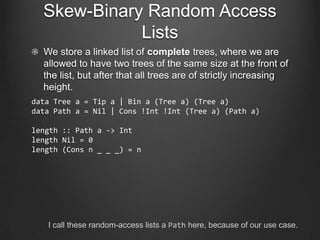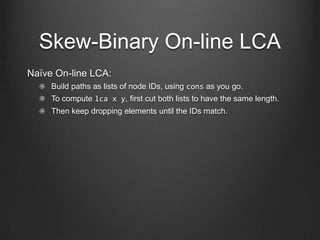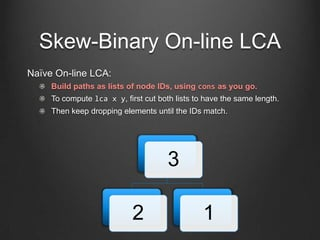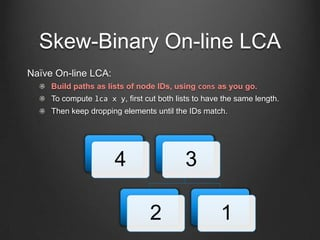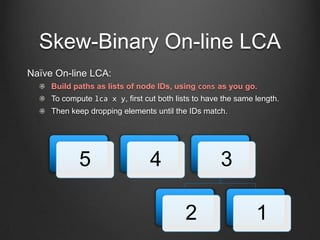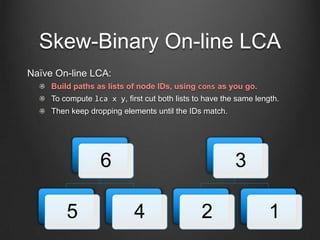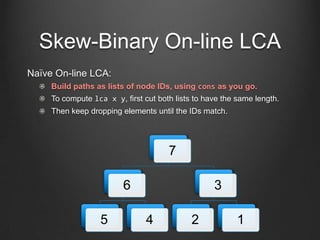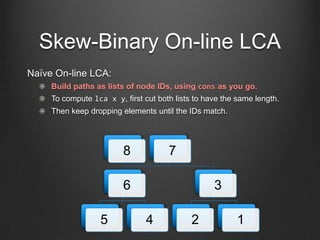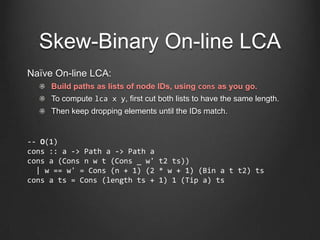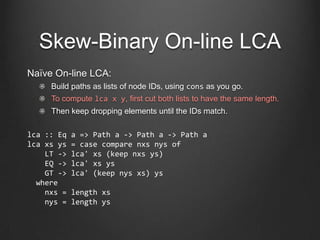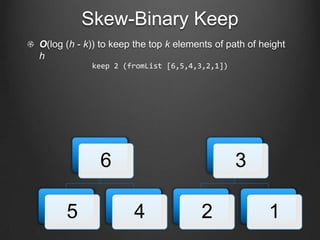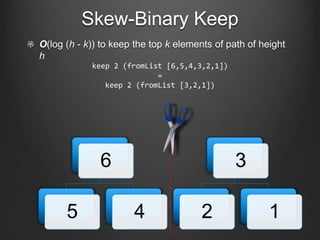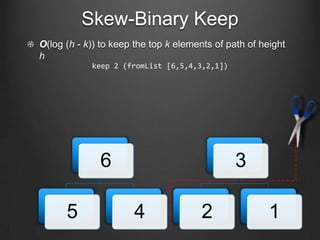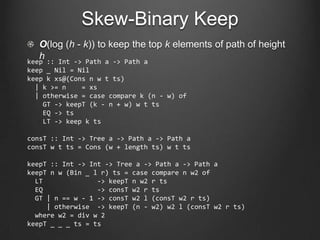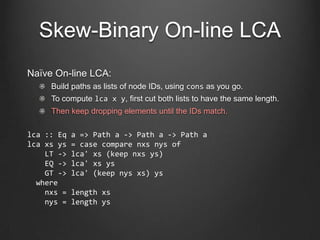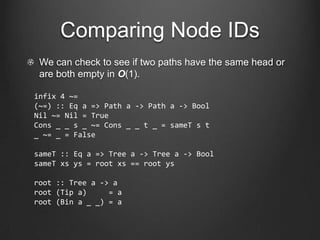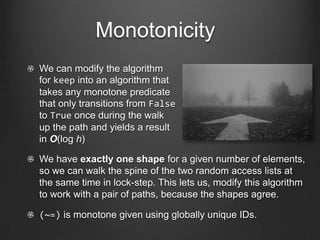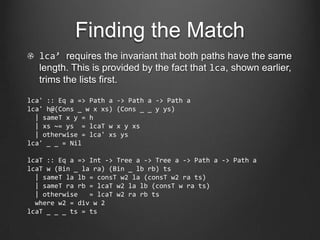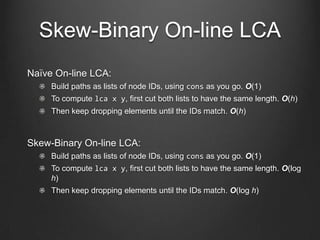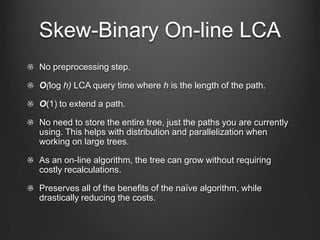1 of 43

### Purely Functional Data Structures for On-Line LCA

1. Purely Functional Data Structures for On-line LCA Edward Kmett
2. Overview The Lowest Common Ancestor (LCA) Problem Tarjan’s Off-line LCA Off-line Tree-Like LCA Off-line Range-Min LCA Naïve On-line LCA Data Structures from Number Systems Skew-Binary Random Access Lists Skew-Binary On-line LCA
3. The Lowest Common Ancestor Problem Given a tree, and two nodes in the tree, find the lowest entry in the tree that is an ancestor to both. A B E C D F G I H J
4. The Lowest Common Ancestor Problem Given a tree and two nodes in the tree, find the lowest entry in the tree that is an ancestor to both. Applications: Computing Dominators in Flow Graphs Three-Way Merge Algorithms in Revision Control Common Word Roots/Suffixes Range-Min Query (RMQ) problems Computing Distance in a Tree …
5. The Lowest Common Ancestor Problem Given a tree and two nodes in the tree, find the lowest entry in the tree that is an ancestor to both. First formalized by Aho, Hopcraft, and Ullman in 1973. They provided ephemeral on-line and off-line versions of the problem in terms of two operations, with their off-line version of the algorithm requiring O(n log*(n)) and their online version requiring O(n log n) steps. Research has largely focused on the off-line versions of this problem where you are given the entire tree a priori.
6. cons, link, or grow? The original formulation of LCA was in terms of two operations link x y which grafts an unattached tree x on as a child of y, and lca x y which computes the lowest common ancestor of x and y. Alternately, we can work with lca x y and cons a y, which returns a new extended version of the path y grown downward with the globally unique node ID a, and We can replace cons a y with a monadic grow y, which tracks the variable supply internally. By using a concurrent variable supply like the one supplied by the concurrent- supply package enables you to grow the tree in parallel.
7. Tarjan’s Off-line LCA In 1979, Robert Tarjan found a way to compute a predetermined set of distinct LCA queries at the same time given the complete tree by creatively using disjoint- set forests in O(nα(n)). (This is stronger condition than the usual offline problem statement.) TarjanOLCA(u) function MakeSet(u); u.ancestor := u; for each v in u.children do TarjanOLCA(v); Union(u,v); Find(u).ancestor := u; u.colour := black; for each v such that {u,v} in P do if v.colour == black print "The LCA of “+u+" and “+v+" is " + Find(v).ancestor;
8. Tarjan’s Off-line LCA In 1979, Robert Tarjan found a way to compute a predetermined set of distinct LCA queries at the same time given the complete tree by creatively using disjoint- set forests in O(nα(n)). In 1983, Harold Gabow and Robert Tarjan improved the asymptotics of the preceding algorithm to O(n) by noting special-case opportunities not available in general purpose disjoint-set forest problems.
9. Tree-Like Off-line LCA In 1984, Dov Harel and Robert Tarjan provided the first asymptotically optimal off-line solution, which converts the tree in O(n) into a structure that can be queried in O(1). In 1988, Baruch Scheiber and Uzi Vishkin simplified that structure, by building arbitrary-fanout trees out of paths and binary trees, and providing fast indexing into each case.
10. Range-Min Off-line LCA In 1993, Omer Berkman and Uzi Vishkin found another conversion with the same O(n) preprocessing using an Euler tour to convert the tree structure into a Range-Min structure, that can be queried in O(1) time. This was improved in 2000 by Michael Bender and Martin Farach-Colton. Alstrup, Gavoille, Kaplan and Rauhe focused on distributing this algorithm. Fischer and Heun reduced the memory requirements, but also show logarithmically slower RMQ algorithms are often faster the common problem sizes of today!
11. Backup Plans
12. Naïve On-line LCA Build paths as lists of node IDs, using cons as you go. x = [5,4,3,2,1] :# 5 y = [6,3,2,1] :# 4 To compute lca x y, first cut both lists to have the same length. x’ = [4,3,2,1], y’ = [6,3,2,1], len = 4 Then keep dropping elements from both until the IDs match. lca x y = [3,2,1] :# 3
13. Naïve On-line LCA No preprocessing step. O(h) LCA query time where h is the length of the path. O(1) to extend a path. No need to store the entire tree, just the paths you are currently using. This helps with distribution and parallelization. As an on-line algorithm, the tree can grow without requiring costly recalculations.
14. Naïve On-line LCA To go faster we’d need to extract a common suffix in sublinear time. Very Well…
15. Data Structures from Number Systems We are already familiar with at least one data structure derived from a number system. data Nat = Zero | Succ Nat data List a = Nil | Cons a (List a) O(1) succ grants us O(1) cons
16. Binary Random-Access Lists We could construct a data structure from binary numbers as well, where you have a linked list of “flags” with 2n elements in them. However, adding 1 to a binary number can affect all log n digits in the number, yielding O(log n) cons.
17. Skew-Binary Numbers 15 7 3 1 0 1 2 1 0 The nth digit has value2n+1-1, and each 1 1 digit has a value of 0,1, or 2. 1 2 2 0 We only allow a single 2 in the 1 0 0 number, which must be the first non-zero 1 0 1 digit. 1 0 2 1 1 0 Every natural number can be uniquely 1 1 1 represented by this scheme. 1 1 2 1 2 0 succ is an O(1) operation. 2 0 0 There are 2n+1-1 nodes in a complete tree 1 0 0 0 of height n.
18. Skew-Binary Random Access Lists We store a linked list of complete trees, where we are allowed to have two trees of the same size at the front of the list, but after that all trees are of strictly increasing height. data Tree a = Tip a | Bin a (Tree a) (Tree a) data Path a = Nil | Cons !Int !Int (Tree a) (Path a) length :: Path a -> Int length Nil = 0 length (Cons n _ _ _) = n I call these random-access lists a Path here, because of our use case.
19. Skew-Binary On-line LCA Naïve On-line LCA: Build paths as lists of node IDs, using cons as you go. To compute lca x y, first cut both lists to have the same length. Then keep dropping elements until the IDs match.
20. Skew-Binary On-line LCA Naïve On-line LCA: Build paths as lists of node IDs, using cons as you go. To compute lca x y, first cut both lists to have the same length. Then keep dropping elements until the IDs match.
21. Skew-Binary On-line LCA Naïve On-line LCA: Build paths as lists of node IDs, using cons as you go. To compute lca x y, first cut both lists to have the same length. Then keep dropping elements until the IDs match. 1
22. Skew-Binary On-line LCA Naïve On-line LCA: Build paths as lists of node IDs, using cons as you go. To compute lca x y, first cut both lists to have the same length. Then keep dropping elements until the IDs match. 2 1
23. Skew-Binary On-line LCA Naïve On-line LCA: Build paths as lists of node IDs, using cons as you go. To compute lca x y, first cut both lists to have the same length. Then keep dropping elements until the IDs match. 3 2 1
24. Skew-Binary On-line LCA Naïve On-line LCA: Build paths as lists of node IDs, using cons as you go. To compute lca x y, first cut both lists to have the same length. Then keep dropping elements until the IDs match. 4 3 2 1
25. Skew-Binary On-line LCA Naïve On-line LCA: Build paths as lists of node IDs, using cons as you go. To compute lca x y, first cut both lists to have the same length. Then keep dropping elements until the IDs match. 5 4 3 2 1
26. Skew-Binary On-line LCA Naïve On-line LCA: Build paths as lists of node IDs, using cons as you go. To compute lca x y, first cut both lists to have the same length. Then keep dropping elements until the IDs match. 6 3 5 4 2 1
27. Skew-Binary On-line LCA Naïve On-line LCA: Build paths as lists of node IDs, using cons as you go. To compute lca x y, first cut both lists to have the same length. Then keep dropping elements until the IDs match. 7 6 3 5 4 2 1
28. Skew-Binary On-line LCA Naïve On-line LCA: Build paths as lists of node IDs, using cons as you go. To compute lca x y, first cut both lists to have the same length. Then keep dropping elements until the IDs match. 8 7 6 3 5 4 2 1
29. Skew-Binary On-line LCA Naïve On-line LCA: Build paths as lists of node IDs, using cons as you go. To compute lca x y, first cut both lists to have the same length. Then keep dropping elements until the IDs match. -- O(1) cons :: a -> Path a -> Path a cons a (Cons n w t (Cons _ w' t2 ts)) | w == w' = Cons (n + 1) (2 * w + 1) (Bin a t t2) ts cons a ts = Cons (length ts + 1) 1 (Tip a) ts
30. Skew-Binary On-line LCA Naïve On-line LCA: Build paths as lists of node IDs, using cons as you go. To compute lca x y, first cut both lists to have the same length. Then keep dropping elements until the IDs match. lca :: Eq a => Path a -> Path a -> Path a lca xs ys = case compare nxs nys of LT -> lca' xs (keep nxs ys) EQ -> lca' xs ys GT -> lca' (keep nys xs) ys where nxs = length xs nys = length ys
31. Skew-Binary Keep O(log (h - k)) to keep the top k elements of path of height h keep 2 (fromList [6,5,4,3,2,1]) 6 3 5 4 2 1
32. Skew-Binary Keep O(log (h - k)) to keep the top k elements of path of height h keep 2 (fromList [6,5,4,3,2,1]) = keep 2 (fromList [3,2,1]) 6 3 5 4 2 1
33. Skew-Binary Keep O(log (h - k)) to keep the top k elements of path of height h keep 2 (fromList [6,5,4,3,2,1]) 6 3 5 4 2 1
34. Skew-Binary Keep O(log (h - k)) to keep the top k elements of path of height h keep :: Int -> Path a -> Path a keep _ Nil = Nil keep k xs@(Cons n w t ts) | k >= n = xs | otherwise = case compare k (n - w) of GT -> keepT (k - n + w) w t ts EQ -> ts LT -> keep k ts consT :: Int -> Tree a -> Path a -> Path a consT w t ts = Cons (w + length ts) w t ts keepT :: Int -> Int -> Tree a -> Path a -> Path a keepT n w (Bin _ l r) ts = case compare n w2 of LT -> keepT n w2 r ts EQ -> consT w2 r ts GT | n == w - 1 -> consT w2 l (consT w2 r ts) | otherwise -> keepT (n - w2) w2 l (consT w2 r ts) where w2 = div w 2 keepT _ _ _ ts = ts
35. Skew-Binary On-line LCA Naïve On-line LCA: Build paths as lists of node IDs, using cons as you go. To compute lca x y, first cut both lists to have the same length. Then keep dropping elements until the IDs match. lca :: Eq a => Path a -> Path a -> Path a lca xs ys = case compare nxs nys of LT -> lca' xs (keep nxs ys) EQ -> lca' xs ys GT -> lca' (keep nys xs) ys where nxs = length xs nys = length ys
36. Comparing Node IDs We can check to see if two paths have the same head or are both empty in O(1). infix 4 ~= (~=) :: Eq a => Path a -> Path a -> Bool Nil ~= Nil = True Cons _ _ s _ ~= Cons _ _ t _ = sameT s t _ ~= _ = False sameT :: Eq a => Tree a -> Tree a -> Bool sameT xs ys = root xs == root ys root :: Tree a -> a root (Tip a) = a root (Bin a _ _) = a
37. Monotonicity We can modify the algorithm for keep into an algorithm that takes any monotone predicate that only transitions from False to True once during the walk up the path and yields a result in O(log h) We have exactly one shape for a given number of elements, so we can walk the spine of the two random access lists at the same time in lock-step. This lets us, modify this algorithm to work with a pair of paths, because the shapes agree. (~=) is monotone given using globally unique IDs.
38. Finding the Match lca’ requires the invariant that both paths have the same length. This is provided by the fact that lca, shown earlier, trims the lists first. lca' :: Eq a => Path a -> Path a -> Path a lca' h@(Cons _ w x xs) (Cons _ _ y ys) | sameT x y = h | xs ~= ys = lcaT w x y xs | otherwise = lca' xs ys lca' _ _ = Nil lcaT :: Eq a => Int -> Tree a -> Tree a -> Path a -> Path a lcaT w (Bin _ la ra) (Bin _ lb rb) ts | sameT la lb = consT w2 la (consT w2 ra ts) | sameT ra rb = lcaT w2 la lb (consT w ra ts) | otherwise = lcaT w2 ra rb ts where w2 = div w 2 lcaT _ _ _ ts = ts
39. Skew-Binary On-line LCA Naïve On-line LCA: Build paths as lists of node IDs, using cons as you go. O(1) To compute lca x y, first cut both lists to have the same length. O(h) Then keep dropping elements until the IDs match. O(h) Skew-Binary On-line LCA: Build paths as lists of node IDs, using cons as you go. O(1) To compute lca x y, first cut both lists to have the same length. O(log h) Then keep dropping elements until the IDs match. O(log h)
40. Skew-Binary On-line LCA No preprocessing step. O(log h) LCA query time where h is the length of the path. O(1) to extend a path. No need to store the entire tree, just the paths you are currently using. This helps with distribution and parallelization when working on large trees. As an on-line algorithm, the tree can grow without requiring costly recalculations. Preserves all of the benefits of the naïve algorithm, while drastically reducing the costs.
41. Now What? We found that skew-binary random access lists can be used to accelerate the naïve online LCA algorithm while retaining the desirable properties. You can install a working version of this algorithm from hackage cabal install lca Next time I’ll talk about the applications of this algorithm to a “revision control” monad which can be used for parallel and incremental computation in Haskell. I am working with Daniel Peebles on a proof of correctness and asymptotic performance in Agda.
42. Any Questions?